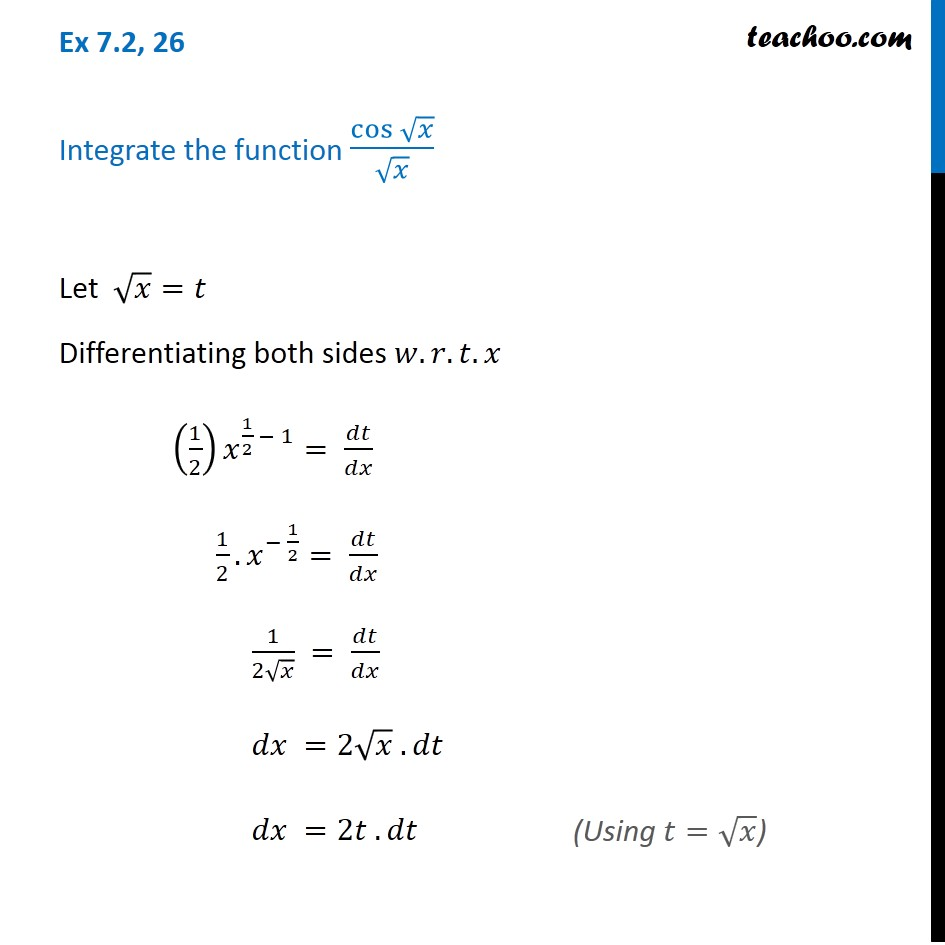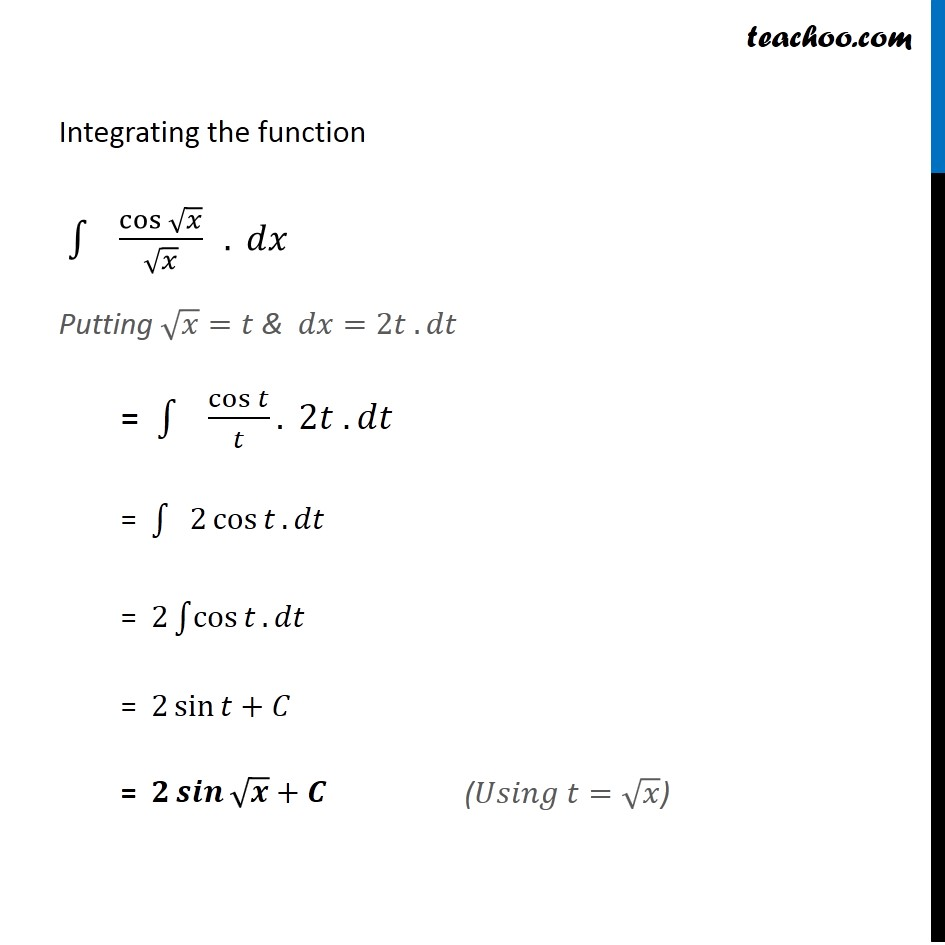1. Chapter 7 Class 12 Integrals (Term 2)
2. Concept wise
3. Integration by substitution - Trignometric - Normal

Transcript

Ex 7.2, 26 Integrate the function cos⁡√𝑥/√𝑥 Let √𝑥=𝑡 Differentiating both sides 𝑤.𝑟.𝑡.𝑥 (1/2) 𝑥^(1/2 − 1)= 𝑑𝑡/𝑑𝑥 1/2. 𝑥^(− 1/2)= 𝑑𝑡/𝑑𝑥 1/(2√𝑥) = 𝑑𝑡/𝑑𝑥 𝑑𝑥 =2√𝑥 . 𝑑𝑡 𝑑𝑥 =2𝑡 . 𝑑𝑡 Integrating the function ∫1▒〖" " cos⁡√𝑥/√𝑥 " " 〗. 𝑑𝑥 Putting √𝑥=𝑡 & 𝑑𝑥=2𝑡 . 𝑑𝑡 = ∫1▒〖" " cos⁡𝑡/𝑡〗. 2𝑡 . 𝑑𝑡 = ∫1▒〖" " 2 cos⁡𝑡 〗. 𝑑𝑡 = 2∫1▒cos⁡𝑡 . 𝑑𝑡 = 2 sin⁡𝑡+𝐶 = 𝟐 𝒔𝒊𝒏⁡√𝒙+𝑪 (𝑈𝑠𝑖𝑛𝑔 𝑡=√𝑥)